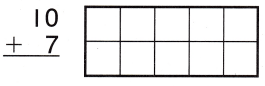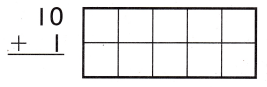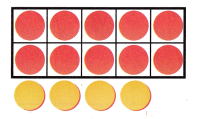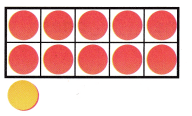Refer to our Texas Go Math Grade 1 Answer Key Pdf to score good marks in the exams. Test yourself by practicing the problems from Texas Go Math Grade 1 Module 3 Assessment Answer Key.

Concept and Skills

Model and draw to solve.
Usesand a ten frame. TEKS 1.3.A

Question 1.Explanation:
10 + 7 = 17
By using a ten frame added 7 to it

Question 2.Explanation:
10 + 1 = 11
By using a ten frame added 1 to it

Write how many tens and ones. Draw to show tens and ones. Write the sum. TEKS 1.3.A

Question 3.
50 + 6 = ___
___ tens __ ones
5 tens 6 ones
Explanation:
5 tens 6 ones is equals to 56

Question 4.
20 + 8 = ___
___ tens __ ones
2 tens and 8 ones
Explanation:
2 tens and 8 ones is equals to 28

Question 5.
80 + 1 = ___
___ tens __ ones
8 tens and 1 ones
Explanation:
8 tens and 1 ones is equals to 81

Question 6.
90 + 9 = ___
___ tens __ ones
9 tens and 9 ones
Explanation:
9 tens and 9 ones is equals to 99

Texas Test Prep

Question 7.
Which number sentence does this model show? TEKS 1.3.A(A) 10 – 4 = 6
(B) 10 + 4 = 14
(C) 5 + 4 = 9
Explanation:
By using the 10 frame added 10 + 4 = 14

Question 8.
Pam has 20 crayons. She gets 6 crayons from a friend. How many crayons does Pam have now? TEKS 1.3.A
(A) 26
(B) 6
(C) 20
Explanation:
20 + 6 = 26
2 tens and 6 ones is equals to 26

Question 9.
What is the sum? TEKS 1.3.A
30 + 3 = ?
(A) 3
(B) 33
(C) 30
Explanation:
30 + 3 = 33
3 tens and 3 ones is equals to 33

Question 10.
Which number sentence does this model show? TEKS 1.3.A(A) 10 + 1 = 11
(B) 10 – 1 = 9
(C) 10 + 5 = 15